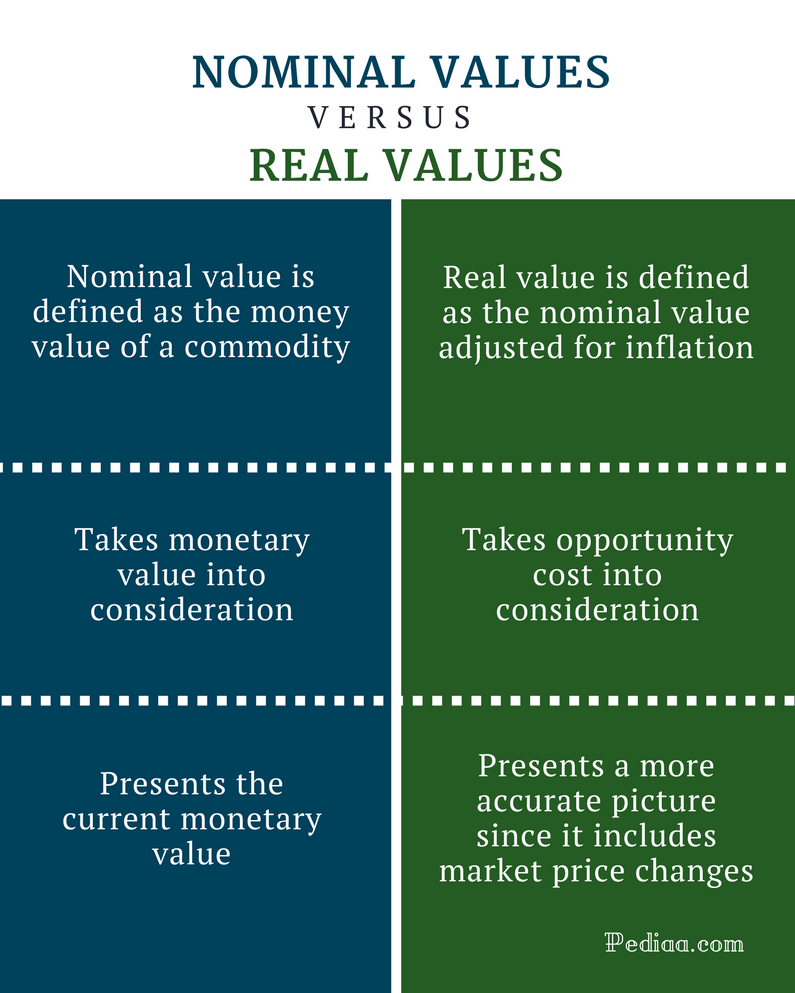# Difference Between Nominal and Real Values

## Main Difference – Nominal vs Real Values

In economics, the value of a given commodity will be measured in two frameworks as nominal value and real value. If a given commodity is expressed in a fixed currency value, it is identified as the nominal value. If the market price changes are added to the nominal value of a particular good or a service, it is known as the real value of that commodity.  Thus, the main difference between nominal and real values is the changes in the market price level. These nominal and real value concepts play a vital role in economics as these two concepts similarly represent in interest rates in the forms of nominal interest rate and real interest rate / GDP etc.

1. What are Nominal Values – Definition, Influencing Factors, Uses

2. What are Real Values  – Definition, Influencing Factors, Uses

3. What is the difference between Nominal and Real Values## What is a Nominal Value

If the economic value of a particular commodity is expressed in terms of a currency value, it is known as the nominal value of that commodity. This is merely a statistical representation; inflation or market price changes are not incorporated in this value. The nominal value provides just an estimation of the price of a particular commodity or bundle of commodities. Thus, it is difficult to make important economic decisions by just looking at these tentative values.## What is a Real Value

If the market price changes are added to the nominal value of a particular good or a service, it is known as the real value of that commodity. Therefore, calculating real value involves adjusting the nominal value according to inflation. Hence, the impact of price movements on the market is removed from the nominal values deflating them with pre-determined economic indices. Therefore, this real value is identified as the most accurate value for the economic decision making.

## Similarities Between Nominal and Real Values

• Both are used to measure the value of commodity prices and can be used to make economic decisions. However, real values are treated as more accurate since it takes market price changes into consideration.
• Both nominal, real concepts are used in different economic grounds such as GDP, output, income, and interest rates.

## Difference Between Nominal and Real Values

### Definition

Nominal value is defined as the money value of a commodity.

Real value is defined as the nominal value adjusted for inflation.

### Opportunity Cost vs Monetary Value

Nominal value takes monetary value into consideration.

Real value takes opportunity cost into consideration.

### Use

Nominal value presents the current money value.

Real value presents a more accurate picture since it includes market price changes (inflation / deflation).

## Nominal vs Real Values – Conclusion

Nominal and real values are two different concepts in Economics. The underlying difference between the two concepts is the changes in market price levels (inflation, deflation). Nominal values (price of goods and services, income, interest rate, income, etc.) are calculated based on the current market values, without taking inflation into consideration. In contrast, real values are adjusted for inflation and are calculated using price indices. Hence, real values always produce a straightforward view of what is really going on in the marketplace behind the price veils.